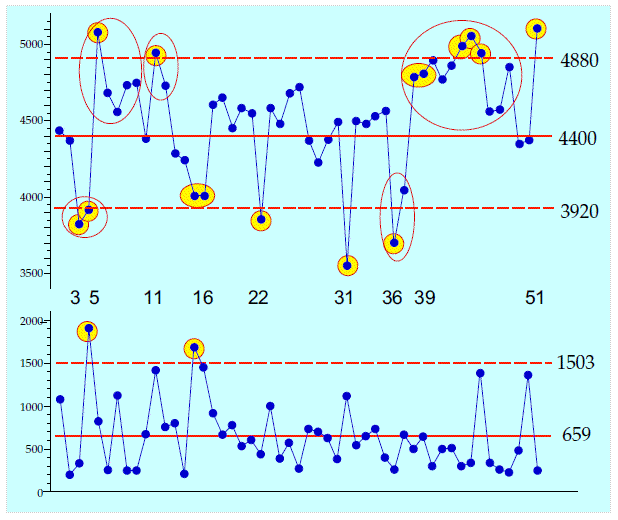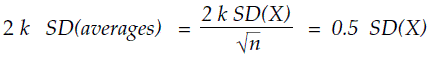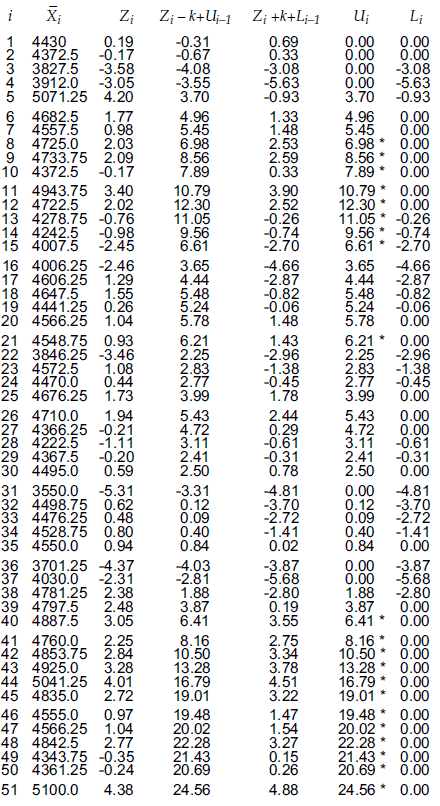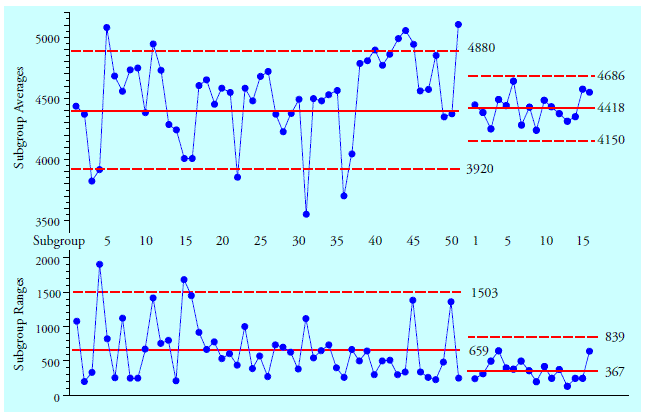#Quality Digest

Featured Product
This Week in Quality Digest Live
Six Sigma Features
Alan Metzel
Introducing the Enhanced Perkin Tracker
Donald J. Wheeler
What you think you know may not be so
William A. Levinson
The AIAG offers a clearly defined and powerful synergy between the three
Donald J. Wheeler
How does it compare with a process behavior chart?
OpusWorks
Eight unique best-practice sessions featuring 11 process improvement and thought leaders
Six Sigma News
Sept. 28–29, 2022, at the MassMutual Center in Springfield, MA
Elsmar Cove is a leading forum for quality and standards compliance
Is the future of quality management actually business management?
Too often process enhancements occur in silos where there is little positive impact on the big picture
Collect measurements, visual defect information, simple Go/No-Go situations from any online device
Good quality is adding an average of 11 percent to organizations’ revenue growth
Floor symbols and decals create a SMART floor environment, adding visual organization to any environment
A guide for practitioners and managers
Making lean Six Sigma easier and adaptable to current workplacesBio

Six Sigma

## The Cumulative Sum Technique

### How does it compare with a process behavior chart?

Published: Monday, October 31, 2022 - 11:03

The cumulative sum (or Cusum) technique is occasionally offered as an alternative to process behavior charts, even though they have completely different objectives. Process behavior charts characterize whether a process has been operated predictably. Cusums assume that the process is already being operated predictably and look for deviations from the target value. Thus, by replacing process characterization with parameter estimation, Cusums beg the very question process behavior charts were created to address.

To illustrate the Cusum approach and compare it with an average chart, I’ll use the example from page 20 of Shewhart’s first book, Economic Control of Quality of Manufactured Product (Martino Fine books, 2015 reprint).These data consist of 204 measurements of electrical resistivity for an insulator. Shewhart organized them into 51 subgroups of size four, based upon the time order in which the measurements were obtained. Figure 1 gives the averages and ranges for these 51 subgroups.Figure 1: Shewhart’s resistivity averages and ranges

The grand average is 4,498 megohms, and the average range is 659 megohms. The target value for this material was 4,400 megohms. If we place the central line for the average chart at 4,400 megohms, our average chart will detect deviations from the target value. Since the objective of Cusum is to detect deviations from target, then using 4,400 as the central line for the average chart will give us a direct comparison between the two techniques.Figure 2: Average and range chart for Shewhart’s data

The average chart shows nine excursions where the measurements were detectably different from 4,400. Including points in the same runs, these excursions involved 27 of the 51 subgroups. The numbers between the two charts denote those subgroups where the average chart first detects each of these nine excursions. Seven of these signals are points outside the three sigma limits, and two signals (at points 16 and 39) are runs beyond two sigma. Thus, the story told by the average chart is one of a process that is off target more than half the time.

Now we turn to see what story a Cusum will tell about these data.

### The cumulative sum technique

Although the original version of the cumulative sum technique was based on a graph, today it is an algorithmic technique. To create your Cusum algorithm, certain information is needed from the data, and certain choices have to be made by the user.

Step one: Select a target value for the time series

The Cusum technique is essentially a sequential test of hypotheses for detecting deviations from a target value. (The null hypothesis being that the process is operating on target.) In the case of the resistivity data, the target value is 4,400 megohms.

Step two: Obtain a measure of dispersion for the time series

Under the assumption that the process has been operated predictably, the usual estimate for dispersion would be the standard deviation statistic computed using the 51 subgroup averages. Here, this statistic is s = 352 megohms. However, since we already know the assumption of a predictable process is not true, we’ll use the average range of 659 megohms to estimate:When a process is operated predictably, these two different estimates of dispersion will be quite similar. By using the second value, we substantially increase the sensitivity of our Cusum technique.

Step three: Compute the standardized deviations from target

We subtract the target value from each subgroup average and divide by Sigma (Averages) to get the standardized deviations from target:Step four: Determine the size of shift to be detected

From 1954 to the present, Cusum has been presented as being more sensitive to process shifts than process behavior charts. One reason for this is that Cusums are traditionally set up to detect small shifts. The Cusum shift constant, k, is one-half the size of the minimum shift that you wish to detect. This shift is expressed as a multiple of the standard deviation of the statistic being tracked by the Cusum. By far, the most common value used for the Cusum shift constant has been k = 0.5. With subgroups of size n = 4, this shift constant will result in a Cusum that should detect shifts greater than:While it is logical to assume that a Cusum that detects shifts greater than 0.5 SD(X) will be more sensitive than the average chart in figure 2, we’ll see this isn't the case.

Step five: Determine the critical value for the cumulative sums

The critical value for the cumulative sums, h, will depend upon the Cusum shift constant, k, and the advertised alpha level for the Cusum technique:Thus, in addition to choosing the size of shift to be detected, the user also has to choose a risk of a false alarm, α. Here, since we are seeking to compare techniques, we will use the nominal alpha level of the average chart, which is α = 0.003. With k = 0.5, the formula above gives:

h = 5.81

Thus, in addition to an estimate of dispersion for the process and a known target value, the Cusum technique depends upon three parameters—k, h, and the subgroup size n.

Step six: Compute the cumulative sums

The Cusum algorithm for the standardized deviations from target computes two partial sums for each value in the time series. These will be the upper cumulative sum, Ui, and the lower cumulative sum, Li, where:

Ui = MAX{ (Zk + Ui–1 ) and 0 }

Li = MIN{ 0 and (Zi+ k + Li–1 ) }

The initial values of U0 and L0 are taken to be zero.

Whenever Ui exceeds the critical sum h, or whenever Li falls below the negative of the critical sum, –h, the Cusum is said to have detected a shift away from the target value.

The operation of the Cusum algorithm is illustrated in the table in figure 3. There, the Cusum identifies three periods involving 21 subgroups where the average is said to be detectably different from the target value of 4,400. These departures were first detected at subgroups 8, 21, and 40.Figure 3: A Cusum algorithm for the average resistivities

The first Cusum signal identifies eight subgroups (subgroups 8 through 15) as being detectably above 4,400. The second Cusum signal identifies subgroup 21 as detectably above 4,400. This subgroup is the fifth in a run of five subgroups that average just over 1 standard error above 4,400 (which is just the size of shift we asked the Cusum to find). Finally, the Cusum identifies the last 12 subgroups (subgroups 40 through 51) as being detectably greater than 4,400.

### Comparison of techniques

How these two techniques worked with this data set is summarized in figure 4. There, we find that these two techniques characterize 18 of the 51 subgroups differently—they disagree on more than one-third of the subgroups!Figure 4: Comparison of average chart and Cusum

So, is the Cusum more sensitive than the average chart? It did detect a one-sigma shift for subgroups 17 through 21 that the average chart did not detect. However, this Cusum missed six three-sigma signals (subgroups 3, 4, 5, 22, 31, and 36), and it also missed two two-sigma signals (subgroups 15 and 16 and subgroups 38 and 39). Moreover, this Cusum reported six subgroups with averages below 4,400 as being detectably greater than 4,400 (subgroups 10, 13, 14, 15, 49, and 50).

So while this Cusum did belatedly detect the two long excursions above 4,400, the inertia inherent in the cumulative sums made the Cusum slow to detect when the deviations began, and slow to detect when those deviations ended. Thus, with eight missed signals and six spurious signals, we can only conclude that the “increased sensitivity” of the Cusum is more of a myth than a reality.

### The rest of the story

The following is what Shewhart wrote about figure 2.

“Several of the [averages] lie outside these limits. This was taken as an indication of the existence of causes of variability which could be found and eliminated.

“Further research was instituted at this point to find these causes of vari­ability. Several were found, and after these had been eliminated an­other series of observed values gave the results [on the right in Figure 5]. Here we see that all of the points lie within the limits. We assumed therefore, upon the basis of this [chart], that it was not feasible for research to go much further in eliminating causes of variability. Because of the impor­tance of this particular experiment, however, considerably more work was done but it failed to reveal causes of variability. Here, then, is a typical case where the [process behavior chart] indicates when variability should be left to chance.”Figure 5: Shewhart’s before-and-after average charts for resistivity

Notice that they not only removed the outlying averages, but they also ended up with much tighter limits centered near 4,400. By identifying the assignable causes of exceptional variation and making them part of the controlled inputs for the process, we not only eliminate outliers, but we also remove major sources of variation from our processes.

### Summary

Because of the many choices required, many different Cusums can be created to meet various criteria. In fact many different Cusums can be created to meet a single criterion. These choices may allow a Cusum to be more sensitive to small shifts than a process behavior chart; however, by the very nature of the inertia inherent in the cumulative sums, they will always be slower than a process behavior chart in detecting large, sudden, or ephemeral changes.

This fact of life has been well documented in the literature. It is the reason several authors have suggested using Shewhart’s average chart along with a Cusum, the idea being that the process behavior chart will detect the large changes while the Cusum will detect the small changes.

Of course, using the Western Electric zone tests with a process behavior chart will accomplish the same thing with much less effort. But when you have invested all the time and effort required to figure out your Cusum algorithm, you are probably going to insist on using it come hell or high water!

Shewhart created process behavior charts to detect large changes because those are the changes where it will be worthwhile to find the cause and fix it. He was not concerned with small shifts because he was looking for ways to improve a process, and small shifts have little to offer.

Cusum was created to maintain the status quo by detecting small shifts in the average of a predictable process. Recent articles show that statisticians are currently trying to tweak the Cusum technique to make it less sensitive to small changes and more sensitive to large changes. This means they are trying to get Cusum to operate more like a process behavior chart.

Why would they try to do this? Because there is a mathematical theorem that tells them that when the process is operated predictably, the Cusum is an optimal technique for detecting sustained shifts in location. Blinded by this limited “optimality,” they look no further. However, when the assumption of a predictable process is not satisfied, the Cusum technique is no longer optimal (as was shown by the example above).

Predictable operation is not a natural state for a production process. And this is the fundamental problem with the Cusum approach. You cannot simply assume a process is being operated predictably. Predictable operation is an accomplishment that can only be maintained by the continued use of a process behavior chart. A process behavior chart is simpler to set up than Cusum; it is easier to use than Cusum; it is more sensitive to shifts that are economically important than is Cusum; and a process behavior chart is necessary to maintain a predictable process. So why go anywhere else?

### Discuss### Donald J. Wheeler

Find out about Dr. Wheeler’s virtual seminars for 2023 at www.spcpress.com. Dr. Wheeler is a fellow of both the American Statistical Association and the American Society for Quality who has taught more than 1,000 seminars in 17 countries on six continents. He welcomes your questions; you can contact him at djwheeler@spcpress.com.

### Thank you for the article in understandable human language.

Thank you for the article, dear Danald

### Process Behavior Chart vs Control Chart

I never miss reading a Dr. Wheeler article in Quality Digest. They are always insightful and challenging.

I understood that Dr. Wheeler uses the term process behavior chart instead of Dr. Shewhart's term control chart. Am I incorrect?

If I am correct, I am thrown off by the use of the material target value of 4,000 for the center line of average chart rather than the grand mean of 4,498. Page 20 of Economic Control of Quality of Manufactured Product used 4,498.

I would appreciate any insight into my misunderstanding.

Thanks

Normally we would use the average of 4498 as the central line.  However, since Cusum is a technique for detecting deviations from a TARGET value, I used the target value of 4,400 on the Average chart to make the comparison between the two twchniques more equitable

### Process Behavior Chart vs Control Chart

Dr. Wheeler, Thanks for clearing that up for me. Your article did say that, but I did not grasp it when I read the article.

Thanks again,

Jonathan

### The Simplest Solution is Often the Best Solution

It's hard enough to get people to use and understand simple X and R charts. Complicating the calculations with other charts makes prospective users run for the hills.

Dr. Wheeler continues to demystify SPC and point us in useful directions. Thanks!

### Wonderful articles

These are just wonderful articles. I used Dr. Wheeler's simple, easy to understand books for some 35 years to bring order out of chaos. Thanks.